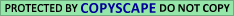## A.5. The Overall Proximity Measures P1, P2, P3 and P4

Let N be the number of word pairs (w, w') in the sample for which the corrected distance c(w, w') is defined (see Sections A.2 and A.3). Let k be the number of such word pairs (w, w') for which c(w, w') <= 1/5.
Define
j, N-j

p1 :=

 N Σ j=k

(
 N j

)
(

 1 --- 5

)
(

 4 --- 5

)

To understand this definition, note that if the c(w, w') were independent random variables that are uniformly distributed over [0,1], then P1 would be the probability that at least k out of N of them are less than are equal to 0.2. However, we do not make or use any such assumptions about uniformity and independence. Thus P1, though calibrated in probability terms, is simply an ordinal index that measures the number of word pairs in a given sample whose words are "pretty close" to each other [i.e., c (w, w') <= 1/5], taking into account the size of the whole sample. It enables us to compare the overall proximity of the word pairs in different samples; specifically, in the samples arising from the different permutations of the 32 personalities.

The statistic P1 ignores all distances c (w, w') greater than 0.2, and gives equal weight to all distances less than 0.2. For a measure that is sensitive to the actual size of the distances, we calculate the product Pc (w, w') over all word pairs (w, w') in the sample. We then define

P2 := FN := (Π c (w, w'),

with N as above, and

FN (X) := X
(
1 - ln X
 (-ln X)2 ------- 2!

+ . . . +
 (-ln X) N-1 ----- (N - 1)!

).

To understand this definition, note first that if x1,x2,...,xn are independent random variables that are uiformly distributed over [0,1], then the distribution of their product X := x1x2 ... xn is given by Prob (X <= X0) =FN (X0); this follows from (3.5) in, since the -ln xi are distributed exponentially, and -ln X = Si (ln xi). The intuition for P2 is then analogous to that for P1: If the c (w, w') were independent random variables that are uniformly distributed over [0,1], then P2 would be the probability that the product P c (w ,w') is as small as it is, or smaller. But as before, we do not use any such uniformity or independence assumptions. Like P1, the statistic P2 is calibrated in probability terms; but rather than thinking of it as a probability, one should think of it simply as an ordinal index that enables us to compare the proximity of the words in word pairs arising from different permutations of the personalities. (FELLER, W. (1966). An Introduction to Probability Theory and Its Applications 2. Wiley, New York.)

We also used two other statistics, P3 and P4. They are defined like P1 and P1, except that for each personality, all appellations starting with the title "Rabbi" are omitted. The reason for considering P3 and P4 is that appellations starting with "Rabbi" often use only the given names of the personality in question. Certain given names are popular and often used (like "John" in English or "Avraham" in Hebrew; thus several different personalities were called Rabbi Avraham. If the phenomenon we are investigating is real, then allowing such appellations might have led to misleadingly low values for c(w,w') when p matches one "Rabbi Avraham" to the dates of another "Rabbi Avraham." This might have resulted in misleadingly low values P1p and P2p for the permuted samples, so in misleadingly low significance levels for P1 and P2 and so, conceivably, to an unjustified rejection of the research hypothesis. Note that this effect is "one-way"; it could not have led to unjustified acceptance of the research hypothesis, since under the null hypothesis the number of Pip exceeding Pi is in any case uniformly distributed. In fact, omitting appellations starting with "Rabbi" did not affect the results substantially (see Table 3); but we could not know this before performing the calculations.

An intuitive feel for the corrected distances (in the original, unpermuted samples) may be gained from Figure 4. Note that in both the first and second samples, the distribution for R looks quite random, whereas for G it is heavily concentrated near 0. It is this concentration that we quantify with the statistics Pi

Click Here To Go To Appendix A.6.